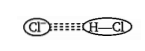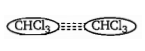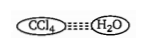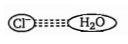Which pair of molecules are polar species?

(a)

(b)

(c)

(d)

High Yielding Test Series + Question Bank - NEET 2020

Difficulty Level:

In which molecule does the chlorine atom has positive partial charge?

(a) HCl

(b) BrCl

(c) $OC{l}_{2}$

(d) $SC{l}_{2}$

High Yielding Test Series + Question Bank - NEET 2020

Difficulty Level:

The incorrect order of lattice energy is:

(a)$Al{F}_{3}>Mg{F}_{2}$          (b) $L{i}_{3}N>L{i}_{2}O$

(c) $NaCl>LiF$           (d)$TiC>ScN$

High Yielding Test Series + Question Bank - NEET 2020

Difficulty Level:

Which of the following set of species have planar structures?

(a)

(b)

(c)

(d)

High Yielding Test Series + Question Bank - NEET 2020

Difficulty Level:

Both $\mathrm{N}{\left({\mathrm{SiH}}_{3}\right)}_{3}$ and $\mathrm{NH}{\left({\mathrm{SiH}}_{3}\right)}_{2}$compounds have trigonal planer skeleton. Incorrect statement about both compound is :

(a) SiNSi bond angle in $\mathrm{NH}{\left({\mathrm{SiH}}_{3}\right)}_{2}$ $>$ SiNSi bond angle in $N{\left({\mathrm{SiH}}_{3}\right)}_{3}$

(b) $\mathrm{N}-\mathrm{Si}$ bond length in $\mathrm{NH}{\left({\mathrm{SiH}}_{3}\right)}_{2}>\mathrm{N}-\mathrm{Si}$ bond length in $\mathrm{N}{\left({\mathrm{SiH}}_{3}\right)}_{3}$

(c) $N-Si$ bond length in $\mathrm{NH}{\left({\mathrm{SiH}}_{3}\right)}_{2} bond length in $\mathrm{N}{\left({\mathrm{SiH}}_{3}\right)}_{3}$

(d) Back bonding strength in $\mathrm{NH}{\left({\mathrm{SiH}}_{3}\right)}_{2}>$ Back bonding strength in $N{\left({\mathrm{SiH}}_{3}\right)}_{3}$

Concept Questions :-

Types of Bonding
High Yielding Test Series + Question Bank - NEET 2020

Difficulty Level:

Which ionic compound has the largest amount of lattice energy?

Concept Questions :-

Lattice/Hydration Energy
High Yielding Test Series + Question Bank - NEET 2020

Difficulty Level:

Which of the following attraction is strongest?

(a)(b)(c)(d)Concept Questions :-

Types of Bonding
High Yielding Test Series + Question Bank - NEET 2020

Difficulty Level:

The incorrect statement regarding $\mathrm{O}{\left({\mathrm{SiH}}_{3}\right)}_{2}$ and ${\mathrm{OCl}}_{2}$ molecule is/are:

(a) The strength of back bonding is more in $\mathrm{O}{\left({\mathrm{SiH}}_{3}\right)}_{2}$ molecule than ${\mathrm{OCl}}_{2}$ molecule

(b) $\mathrm{Si}—\mathrm{O}—\mathrm{Si}$ bond angle in $\mathrm{O}{\left({\mathrm{SiH}}_{3}\right)}_{2}$ is greater than $\mathrm{Cl}—\mathrm{O}—\mathrm{Cl}$ bond angle in ${\mathrm{OCl}}_{2}$

(c) The nature of back bond in both molecules is $2{\mathrm{p}}_{\sigma }‐3{\mathrm{d}}_{\mathrm{\pi }}$

(d) Hybridisation of central $\mathrm{O}‐\mathrm{atom}$ in both molecules is same

Concept Questions :-

Hybridisation
High Yielding Test Series + Question Bank - NEET 2020

Difficulty Level:

Which of the following compound have the same no. of lone pair with their central atom?

(a)             (b) ${\mathrm{BrF}}_{3}$                 (c) ${\mathrm{XeF}}_{2}$                     (d) ${\mathrm{H}}_{3}{\mathrm{S}}^{+}$

(e) Triple Methylene

options are as follows :

(a) (iv) and (v)                                     (b) (i) and (iii)

(c) (i) and (ii)                                       (d) (ii) (iv), (v)

Concept Questions :-

Hybridisation
High Yielding Test Series + Question Bank - NEET 2020

Difficulty Level:

Which of the following order of molecular force of attraction among given species is incorrect?

(a) HI > HBr > $C{l}_{2}$

(b) $C{H}_{3}Cl>C{l}_{2}>CC{l}_{4}>C{H}_{4}$

(c) n- pentane > iso-pentane > neo-pentane

(d) $O{H}_{2}>O{\left(C{H}_{3}\right)}_{2}>OB{r}_{2}$

Concept Questions :-

Polarity